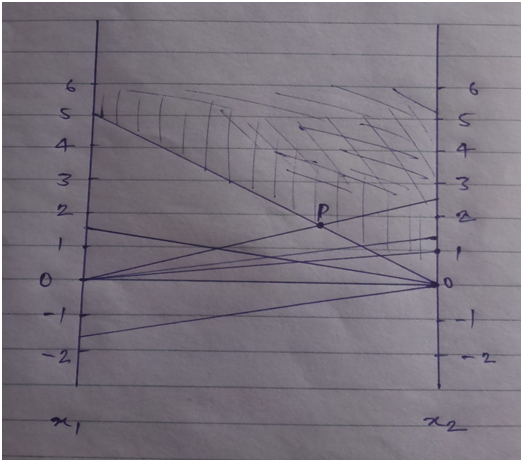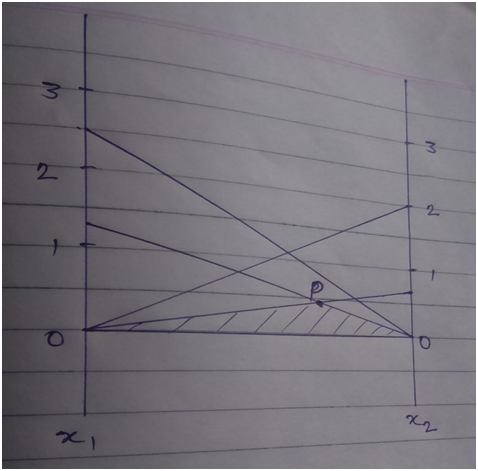# Using the graphical method to find the feasible point-69705

6.

a.   max. z = 5x1 + 6x2

s.t.   -2x1 + 3x2 = 3   ………………i

x1 + 2x2 <= 5  ………………ii

6x1 + 7x2 <= 3 …………….iii

For eqn   i  if x1 = 0 ; x2 = 1

x2 = 0 ; x1 = -3/2

ii  if x1 = 0 ; x2 = 5/2

x2 = 0 ; x1 = 5

iii if   x1 = 0 ; x2 = 3/7

x2 = 0 ; x1 = ½

Using the graphical method to find the feasible pointSo the feasible solution will be-

Max. z =  5x1 + 6x2

= 5*5 + 6*5/2

= 40

b.   min. z =    5x1 + 6x2

s.t. 4x1 + 5x2 > = 10  ………………..i

4x1 + 8x2 > = 5  ………………….ii

For i if x1 = 0 ; x2 = 2

x2 = 0 ; x1 = 5/2

ii  x1 = 0 ; x2 = 5/8

x2 = 0 ; x1 = 5/4

Applying the graphical method to find the feasible point PThe feasible solution will be about point P and the values of x1 and x2 about this point are x1 = 5/4  and x2 = 5/8

So the feasible solution will be

min. z =  5x1 + 6x2

=  5*5/4 + 6*5/8

= 10

7.   Given that min. z = 5x1 + 6x2 + 3x3

s.t. 5x1 + 5x2 + 3x3 >= 50  …………….i

x1 + x2 – x3 >= 20  ………………….ii

7x1 + 6x2 – 9x3 >= 30  ……………..iii

5x1 + 5x2 + 5x3 >= 35  …………….iv

2x1 + 4x2 – 15x3 >= 10  …………….v

12x1 + 10x2 >= 90  …………………..vi

x2 – 10x3 >=  20  ……………………vii

x1 , x2 , x3 >= 0

I will use the dual method to solve the given linear programming problem  as in the dual method we can easily find the dual point and will not be a lengthy procedure.